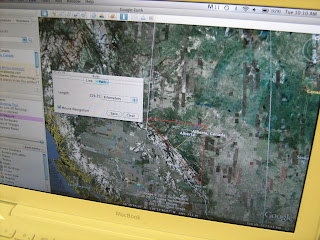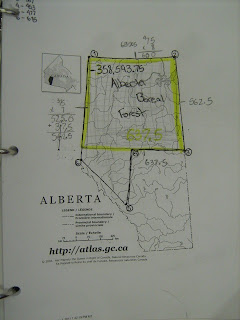Blog has moved, redirecting... ...

Boreal Math Part 2Recently we shared some posts about a grade 6 math problem built around the question: Are there enough trees in Canada's Boreal Forest to be considered the lungs of the Earth?

In order to solve this problem, students were given a few pieces of background information and worked in small groups to formulate the specific calculations they'd need to solve to answer the question.

One of the two grade 6 teachers, Erin Couillard, has just completed a second portion of the Boreal Forest problem this time answering the question: Does the Boreal Forest in Alberta produce enough oxygen to be the lungs of Alberta?

The reason Erin wanted to do a second problem was much of the first question was solved in small groups, and she wanted to see how the students would fare working through a second, quite similar question, this time more on individually.The other piece that was different this second time was the inclusion of area calculations. In order to solve the problem the first calculation that students had to complete was to determine the size of Alberta's Boreal Forest. The nice thing about the location of the Boreal Forest in Alberta is that forms a shape that is easy to get a rough estimate of the size.

The first step was to get the longitude and latitude of some of the key points around the perimeter of Alberta's Boreal Forest using a site called Databasin. Databasin is a very powerful and free GIS website that lets the user layer a variety of different data sets on top of maps.

Using this tool made it easy to get a number of longitude and latitude points for the forest.

Next these points were given to students. In Google Earth the students were able to plot these points and then use the ruler tool to determine the different lengths around the perimeter of the Boreal forest. While most students used Google Earth, a few chose to use paper maps and their scales.As students began to work through the area calculations, it was interesting to see the different ways students solved the problems. After breaking the entire forest into its component shapes, students began to wrestle with how to determine the most accurate area for the shapes.

For example, when calculating the area of the rectangular-like shape that makes up most of Alberta's Boreal Forest,
different students approached this in different ways. Some took the basic approach of calculating the area as a square, length times width. Other students realized the sides were all different lengths, so they multiplied the average of the length times the average of the width. Still others broke the rectangle into two separate triangles, using the different lengths and width.

In this video you'll see students working through the problem and explaining some of their mathematical thinking. You'll also see how Google Earth can be embedded into mathematical problems:

For the assessment component, students were asked to create a short podcast explaining their solutions. This was another change from part one of the project. Students had also been asked to create podcast explanations for part 1, but they were often too long and unrefined. To improve the podcasts, Erin worked with her students to co-create some criteria for an effective podcast, then had her students review their old ones and make suggestions for improvement. There was a noticeable improvement in the second round of podcasts.

You can view one for the podcasts here:

Here's the rubric Erin designed for the final student podcast: